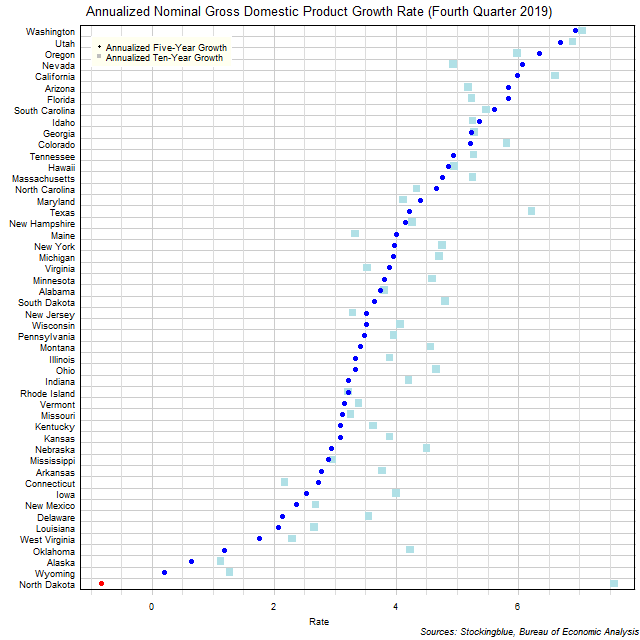# Long-Term US GDP Growth Rate by State, Fourth Quarter 2019

Jun 24, 2020The chart above shows the annualized nominal gross domestic product (GDP) growth rate in each US state over the past five years as of the fourth quarter of 2019 and the growth rate over the past ten years.  Only one state experienced negative growth in either time period.

## Findings

• The difference between the state with the largest annualized five-year growth rate, Washington, and the state with the smallest, North Dakota, is 7.77 percentage points.
• The difference between the state with the largest annualized ten-year growth rate, North Dakota, and the state with the smallest, Alaska, is 6.44 percentage points.
• The median annualized five-year growth rate in the 50 US states is 3.58% and the mean 3.74%.
• The median annualized ten-year growth rate in the 50 US states is 4.25% and the mean 4.33%.
• Thirteen states' economies grew faster over the past five years than they did over the past ten years.  No state had the same annualized growth rate over both periods.  The remaining 37 states saw their economies grow slower over the past five years than they did over the past ten years.

## Caveats

• Data is from the fourth quarters of 2009, 2014, and 2019.
• The data is seasonally adjusted in current dollars.
• Growth rates may differ from those provided by the Bureau of Economic Analysis as the BEA's growth rates are based on chained dollars in conjunction with the chain index or the quality index for real GDP.
• All figures are rounded to the nearest hundredth.

## Details

North Dakota had the largest decrease over the past five years with an annualized growth rate of -0.83%.  Washington had the largest growth with an annualized gain of 6.94%.

Over the past ten years, Alaska had the smallest increase with an annualized growth rate of 1.13% while North Dakota had the largest growth with an annualized growth rate of 7.57%.

North Dakota saw the largest decrease in its growth rate between its annualized ten-year growth and its annualized five-year growth slowing its rate by 8.40 percentage points.  Nevada had the largest increase in its growth rate between its annualized ten-year growth and its annualized five-year growth ramping up its rate by 1.12 percentage points.

Maine went from having the 40th largest annualized growth rate over the past ten years to the 19th largest annualized growth rate over the past five years.  Conversely, North Dakota went from having the highest annualized growth rate over the past ten years to the 50th highest annualized growth rate over the past five years.  Only three states had annualized growth rates in the top five both over the past ten years and over the past five years: Washington (2nd highest annualized growth over ten years, highest annualized growth over five years), Utah (3rd highest ten-year, 2nd highest five-year), and California (4th highest ten-year, 5th highest five-year).  Four states have ranked in the bottom five over both periods: Louisiana (46th ten-year, 45th five-year), West Virginia (47th ten-year, 46th five-year), Alaska (50th ten-year, 48th five-year), and Wyoming (49th ten-year, 49th five-year).

## Sources

US Bureau of Economic Analysis.  2020.  "GDP by State."  Accessed May 27, 2020.  https://www.bea.gov/data/gdp/gdp-state.

Filed under: Charts and Graphs